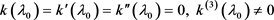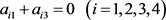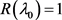﻿ 某耦合边界条件下四阶常微分算子特征值的秩

# 某耦合边界条件下四阶常微分算子特征值的秩The Rank of Eigenvalue of a Four-Order Ordinary Differential Operator under Coupled Boundary Condition

Abstract: In this paper, a class of four-order ordinary differential operators with coupled boundary conditions is discussed. By using the Lagrange equation of the operator's corresponding formula, the relation between the rank of the eigenvalue of the problem and the zero point weight of an entire function is obtained.

1. 问题的提出

$L\equiv -\frac{{\text{d}}^{4}}{\text{d}{x}^{4}}+q\left(x\right)$$x\in \left(0,1\right)$$q\left(x\right)\in C\left[0,1\right]$ 。考虑以下耦合边界条件下的特征值问题：

$Ly=-{y}^{\left(4\right)}+q\left(x\right)y=\lambda y$ (1)

$y\left(0\right)={y}^{\prime }\left(1\right),{y}^{\prime }\left(0\right)={y}^{″}\left(1\right),{y}^{″}\left(0\right)={y}^{\left(3\right)}\left(1\right),{y}^{\left(3\right)}\left(0\right)=y\left(1\right)$ (2)

${Q}_{i}\left(x,\lambda \right)$ $\left(i=1,2,3,4\right)$ 是四个满足(1)式的解，它们分别在 $x=0$ 处满足

$\left(\begin{array}{c}{Q}_{1}\left(0,\lambda \right)\\ {{Q}^{\prime }}_{1}\left(0,\lambda \right)\\ {{Q}^{″}}_{1}\left(0,\lambda \right)\\ {Q}_{1}^{\left(3\right)}\left(0,\lambda \right)\end{array}\right)=\left(\begin{array}{c}1\\ 0\\ 0\\ 0\end{array}\right),\text{}\left(\begin{array}{c}{Q}_{2}\left(0,\lambda \right)\\ {{Q}^{\prime }}_{2}\left(0,\lambda \right)\\ {{Q}^{″}}_{2}\left(0,\lambda \right)\\ {Q}_{2}^{\left(3\right)}\left(0,\lambda \right)\end{array}\right)=\left(\begin{array}{c}0\\ 1\\ 0\\ 0\end{array}\right)$ ,

$\left(\begin{array}{c}{Q}_{3}\left(0,\lambda \right)\\ {{Q}^{\prime }}_{3}\left(0,\lambda \right)\\ {{Q}^{″}}_{3}\left(0,\lambda \right)\\ {Q}_{3}^{\left(3\right)}\left(0,\lambda \right)\end{array}\right)=\left(\begin{array}{c}0\\ 0\\ 1\\ 0\end{array}\right),\text{}\left(\begin{array}{c}{Q}_{4}\left(0,\lambda \right)\\ {{Q}^{\prime }}_{4}\left(0,\lambda \right)\\ {{Q}^{″}}_{4}\left(0,\lambda \right)\\ {Q}_{4}^{\left(3\right)}\left(0,\lambda \right)\end{array}\right)=\left(\begin{array}{c}0\\ 0\\ 0\\ 1\end{array}\right)$ ,

$y\left(x\right)=\underset{i=1}{\overset{4}{\sum }}{c}_{i}{Q}_{i}\left(x,{\lambda }_{0}\right)$ 是问题(1) (2)的特征函数，其中 ${\lambda }_{0}$ 是特征值，则 $y\left(x\right)$ 满足(2)式，即：

$\left\{\begin{array}{c}{c}_{1}={c}_{1}{{Q}^{\prime }}_{1}\left(1,{\lambda }_{0}\right)+{c}_{2}{{Q}^{\prime }}_{2}\left(1,{\lambda }_{0}\right)+{c}_{3}{{Q}^{\prime }}_{3}\left(1,{\lambda }_{0}\right)+{c}_{4}{{Q}^{\prime }}_{4}\left(1,{\lambda }_{0}\right)\\ {c}_{2}={c}_{1}{{Q}^{″}}_{1}\left(1,{\lambda }_{0}\right)+{c}_{2}{{Q}^{″}}_{2}\left(1,{\lambda }_{0}\right)+{c}_{3}{{Q}^{″}}_{3}\left(1,{\lambda }_{0}\right)+{c}_{4}{{Q}^{″}}_{4}\left(1,{\lambda }_{0}\right)\\ {c}_{3}={c}_{1}{{Q}^{‴}}_{1}\left(1,{\lambda }_{0}\right)+{c}_{2}{{Q}^{‴}}_{2}\left(1,{\lambda }_{0}\right)+{c}_{3}{{Q}^{‴}}_{3}\left(1,{\lambda }_{0}\right)+{c}_{4}{{Q}^{‴}}_{4}\left(1,{\lambda }_{0}\right)\\ {c}_{4}={c}_{1}{Q}_{1}\left(1,{\lambda }_{0}\right)+{c}_{2}{Q}_{2}\left(1,{\lambda }_{0}\right)+{c}_{3}{Q}_{3}\left(1,{\lambda }_{0}\right)+{c}_{4}{Q}_{4}\left(1,{\lambda }_{0}\right)\end{array}$ (3)

$k\left({\lambda }_{0}\right)=|\begin{array}{cccc}{{Q}^{\prime }}_{1}\left(1,{\lambda }_{0}\right)-1& {{Q}^{″}}_{1}\left(1,{\lambda }_{0}\right)& {Q}_{1}^{\left(3\right)}\left(1,{\lambda }_{0}\right)& {Q}_{1}\left(1,{\lambda }_{0}\right)\\ {{Q}^{\prime }}_{2}\left(1,{\lambda }_{0}\right)& {{Q}^{″}}_{2}\left(1,{\lambda }_{0}\right)-1& {Q}_{2}^{\left(3\right)}\left(1,{\lambda }_{0}\right)& {Q}_{2}\left(1,{\lambda }_{0}\right)\\ {{Q}^{\prime }}_{3}\left(1,{\lambda }_{0}\right)& {{Q}^{″}}_{3}\left(1,{\lambda }_{0}\right)& {Q}_{3}^{\left(3\right)}\left(1,{\lambda }_{0}\right)-1& {Q}_{3}\left(1,{\lambda }_{0}\right)\\ {{Q}^{\prime }}_{4}\left(1,{\lambda }_{0}\right)& {{Q}^{″}}_{4}\left(1,{\lambda }_{0}\right)& {Q}_{4}^{\left(3\right)}\left(1,{\lambda }_{0}\right)& {Q}_{4}\left(1,{\lambda }_{0}\right)-1\end{array}|$ (4)i) ${\lambda }_{0}$ 为问题(1)(2)的特征值 $⇔k\left({\lambda }_{0}\right)=0$

ii) $R\left({\lambda }_{0}\right)+R\left(\Omega \left({\lambda }_{0}\right)\right)=4$

$\left(\begin{array}{c}{H}_{1}\left(x,\lambda \right)\\ {H}_{2}\left(x,\lambda \right)\\ {H}_{3}\left(x,\lambda \right)\\ {H}_{4}\left(x,\lambda \right)\end{array}\right)=A\left(\begin{array}{c}{Q}_{1}\left(x,\lambda \right)\\ {Q}_{2}\left(x,\lambda \right)\\ {Q}_{3}\left(x,\lambda \right)\\ {Q}_{4}\left(x,\lambda \right)\end{array}\right),\text{}A=\left(\begin{array}{cccc}{a}_{11}& {a}_{12}& {a}_{13}& {a}_{14}\\ {a}_{21}& a{}_{22}& {a}_{23}& {a}_{24}\\ {a}_{31}& {a}_{32}& {a}_{33}& {a}_{34}\\ {a}_{41}& {a}_{42}& {a}_{43}& {a}_{44}\end{array}\right)$ . (5)

${T}_{i}={\left({a}_{i1},{a}_{i2},{a}_{i3},{a}_{i4}\right)}^{Τ}$$i=1,2,3,4$ ，其中 ${T}_{i}\ne 0$ 。如果 $k\left({\lambda }_{0}\right)=0$${\Omega }^{Τ}\left(\lambda \right){T}_{i}=0$ ，则：

i) ${H}_{i}\left(x,{\lambda }_{0}\right)$ 是问题(1)(2)的特征函数，则满足

$\left\{\begin{array}{l}{H}_{i}\left(0,{\lambda }_{0}\right)={{H}^{\prime }}_{i}\left(1,{\lambda }_{0}\right)={a}_{i1}\hfill \\ {{H}^{\prime }}_{i}\left(0,{\lambda }_{0}\right)={{H}^{″}}_{i}\left(1,{\lambda }_{0}\right)={a}_{i2}\hfill \\ {{H}^{″}}_{i}\left(0,{\lambda }_{0}\right)={H}_{i}^{\left(3\right)}\left(1,{\lambda }_{0}\right)={a}_{i3}\hfill \\ {H}_{i}^{\left(3\right)}\left(0,{\lambda }_{0}\right)={H}_{i}\left(1,{\lambda }_{0}\right)={a}_{i4}\hfill \end{array}\text{}\left(i=1,2,3,4\right)$

ii) ${H}_{1},{H}_{2},{H}_{3},{H}_{4}$ 的极大无关组个数为 $R\left(A\right)$

${\int }_{a}^{b}g\left(Lf\right)\text{d}x-{\int }_{a}^{b}f\left(Lg\right)\text{d}x={\left[|\begin{array}{cc}f& g\\ {f}^{‴}& {g}^{‴}\end{array}|-|\begin{array}{cc}{f}^{\prime }& {g}^{\prime }\\ {f}^{″}& {g}^{″}\end{array}|\right]}_{a}^{b}$

$\Omega \left({\lambda }_{0}\right)B=\left(\begin{array}{cccc}-{a}_{12}-{a}_{14}& -{a}_{22}-{a}_{24}& -{a}_{32}-{a}_{34}& -{a}_{42}-{a}_{44}\\ {a}_{11}+{a}_{13}& {a}_{21}+{a}_{23}& {a}_{31}+{a}_{33}& {a}_{41}+{a}_{43}\\ -{a}_{12}-{a}_{14}& -{a}_{22}-{a}_{24}& -{a}_{32}-{a}_{34}& -{a}_{42}-{a}_{44}\\ {a}_{11}+{a}_{13}& {a}_{21}+{a}_{23}& {a}_{31}+{a}_{33}& {a}_{41}+{a}_{43}\end{array}\right)$

$B=\left(\begin{array}{cccc}{a}_{12}& {a}_{22}& {a}_{32}& {a}_{42}\\ -{a}_{11}& -{a}_{21}& -{a}_{31}& -{a}_{41}\\ {a}_{14}& {a}_{24}& {a}_{34}& {a}_{44}\\ -{a}_{13}& -{a}_{23}& -{a}_{33}& -{a}_{43}\end{array}\right)$

$\begin{array}{c}0={\int }_{0}^{1}{Q}_{j}\left(x,{\lambda }_{0}\right)\left(L{H}_{i}\left(x,{\lambda }_{0}\right)\right)\text{d}x-{\int }_{0}^{1}\left(L{Q}_{j}\left(x,{\lambda }_{0}\right)\right){H}_{i}\left(x,{\lambda }_{0}\right)\text{d}x\\ ={\left[|\begin{array}{cc}{H}_{i}\left(x,{\lambda }_{0}\right)& {Q}_{j}\left(x,{\lambda }_{0}\right)\\ {H}_{i}^{\left(3\right)}\left(x,{\lambda }_{0}\right)& {Q}_{j}^{\left(3\right)}\left(x,{\lambda }_{0}\right)\end{array}|-|\begin{array}{cc}{{H}^{\prime }}_{i}\left(x,{\lambda }_{0}\right)& {{Q}^{\prime }}_{j}\left(x,{\lambda }_{0}\right)\\ {{H}^{″}}_{i}\left(x{\lambda }_{0}\right)& {{Q}^{″}}_{j}\left(x,{\lambda }_{0}\right)\end{array}|\right]}_{0}^{1}\\ ={H}_{i}\left(1,{\lambda }_{0}\right){Q}_{j}^{\left(3\right)}\left(1,{\lambda }_{0}\right)-{H}_{i}^{\left(3\right)}\left(1,{\lambda }_{0}\right){Q}_{j}\left(1,{\lambda }_{0}\right)-{H}_{i}\left(0,{\lambda }_{0}\right){Q}_{j}^{\left(3\right)}\left(0,{\lambda }_{0}\right)+{H}_{i}^{\left(3\right)}\left(0,{\lambda }_{0}\right){Q}_{j}\left(0,{\lambda }_{0}\right)\\ \text{}-{{H}^{\prime }}_{i}\left(1,{\lambda }_{0}\right){{Q}^{″}}_{j}\left(1,{\lambda }_{0}\right)+{{H}^{″}}_{i}\left(1,{\lambda }_{0}\right){{Q}^{\prime }}_{j}\left(1,{\lambda }_{0}\right)+{{H}^{\prime }}_{i}\left(0,{\lambda }_{0}\right){{Q}^{″}}_{j}\left(0,{\lambda }_{0}\right)-{{H}^{″}}_{i}\left(0,{\lambda }_{0}\right){{Q}^{\prime }}_{j}\left(0,{\lambda }_{0}\right)\end{array}$

$\begin{array}{l}={a}_{i4}{Q}_{j}^{\left(3\right)}\left(1,{\lambda }_{0}\right)-{a}_{i3}{Q}_{j}\left(1,{\lambda }_{0}\right)-{a}_{i1}{Q}_{j}^{\left(3\right)}\left(0,{\lambda }_{0}\right)+{a}_{i4}{Q}_{j}\left(0,{\lambda }_{0}\right)-{a}_{i1}{{Q}^{″}}_{j}\left(1,{\lambda }_{0}\right)\\ \text{}+{a}_{i2}{{Q}^{\prime }}_{j}\left(1,{\lambda }_{0}\right)+{a}_{i2}{{Q}^{″}}_{j}\left(0,{\lambda }_{0}\right)-{a}_{i3}{{Q}^{\prime }}_{j}\left(0,{\lambda }_{0}\right)\end{array}$

$〈{H}_{i},{H}_{j}〉={\int }_{0}^{1}{H}_{i}\left(x\right){H}_{j}\left(x\right)\text{d}x$ ,

$\begin{array}{l}\left(\begin{array}{cccc}〈{H}_{1},{H}_{1}〉& 〈{H}_{1},{H}_{2}〉& 〈{H}_{1},{H}_{3}〉& 〈{H}_{1},{H}_{4}〉\\ 〈{H}_{2},{H}_{1}〉& 〈{H}_{2},{H}_{2}〉& 〈{H}_{2},{H}_{3}〉& 〈{H}_{2},{H}_{4}〉\\ 〈{H}_{3},{H}_{1}〉& 〈{H}_{3},{H}_{2}〉& 〈{H}_{3},{H}_{3}〉& 〈{H}_{3},{H}_{4}〉\\ 〈{H}_{4},{H}_{1}〉& 〈{H}_{4},{H}_{2}〉& 〈{H}_{4},{H}_{3}〉& 〈{H}_{4},{H}_{4}〉\end{array}\right)\\ =\left(\begin{array}{cccc}{{\stackrel{˙}{H}}^{\prime }}_{1}& {{\stackrel{˙}{H}}^{″}}_{1}& {\stackrel{˙}{H}}_{1}^{\left(3\right)}& {\stackrel{˙}{H}}_{1}\\ {{\stackrel{˙}{H}}^{\prime }}_{2}& {\stackrel{˙}{{{H}^{\prime }}^{\prime }}}_{2}& {\stackrel{˙}{H}}_{2}^{\left(3\right)}& {\stackrel{˙}{H}}_{2}\\ {{\stackrel{˙}{H}}^{\prime }}_{3}& {\stackrel{˙}{{{H}^{\prime }}^{\prime }}}_{3}& {\stackrel{˙}{H}}_{3}^{\left(3\right)}& {\stackrel{˙}{H}}_{3}\\ {{\stackrel{˙}{H}}^{\prime }}_{4}& {\stackrel{˙}{{{H}^{\prime }}^{\prime }}}_{4}& {\stackrel{˙}{H}}_{4}^{\left(3\right)}& {\stackrel{˙}{H}}_{4}\end{array}\right)\left(\begin{array}{cccc}-{a}_{12}& -{a}_{22}& -{a}_{32}& -{a}_{42}\\ {a}_{11}& {a}_{21}& {a}_{31}& {a}_{41}\\ -{a}_{14}& -{a}_{24}& -{a}_{34}& -{a}_{44}\\ {a}_{13}& {a}_{23}& {a}_{33}& {a}_{43}\end{array}\right)\end{array}$

$\begin{array}{c}〈{H}_{i},{H}_{j}〉=\underset{\lambda \to {\lambda }_{0}}{\mathrm{lim}}\frac{1}{\lambda -{\lambda }_{0}}\left(\lambda -{\lambda }_{0}\right){\int }_{0}^{1}{H}_{i}\left(x,\lambda \right){H}_{j}\left(x,{\lambda }_{0}\right)\text{d}x\\ =\underset{\lambda \to {\lambda }_{0}}{\mathrm{lim}}\frac{1}{\lambda -{\lambda }_{0}}\left[{\int }_{0}^{1}{H}_{j}\left(x,{\lambda }_{0}\right)\left(L{H}_{i}\left(x,\lambda \right)\right)\text{d}x-{\int }_{0}^{1}{H}_{i}\left(x,\lambda \right)\left(L{H}_{j}\left(x,{\lambda }_{0}\right)\right)\text{d}x\right]\\ =\underset{\lambda \to {\lambda }_{0}}{\mathrm{lim}}\frac{1}{\lambda -{\lambda }_{0}}\left\{{|\begin{array}{cc}{H}_{i}\left(x,\lambda \right)& {H}_{j}\left(x,{\lambda }_{0}\right)\\ {H}_{i}^{\left(3\right)}\left(x,\lambda \right)& {H}_{j}^{\left(3\right)}\left(x,{\lambda }_{0}\right)\end{array}|}_{0}^{1}-{|\begin{array}{cc}{{H}^{\prime }}_{i}\left(x,\lambda \right)& {{H}^{\prime }}_{j}\left(x,{\lambda }_{0}\right)\\ {{H}^{″}}_{i}\left(x,\lambda \right)& {{H}^{″}}_{j}\left(x,{\lambda }_{0}\right)\end{array}|}_{0}^{1}\right\}\end{array}$

$\begin{array}{l}=\underset{\lambda \to {\lambda }_{0}}{\mathrm{lim}}\frac{1}{\lambda -{\lambda }_{0}}\left\{\left[{H}_{i}\left(1,\lambda \right){{H}^{‴}}_{j}\left(1,{\lambda }_{0}\right)-{H}_{j}\left(1,{\lambda }_{0}\right){{H}^{‴}}_{i}\left(1,\lambda \right)-{H}_{i}\left(0,\lambda \right){{H}^{‴}}_{j}\left(0,{\lambda }_{0}\right)+{H}_{j}\left(0,{\lambda }_{0}\right){{H}^{‴}}_{i}\left(0,\lambda \right)\right]\\ \text{}+\left[-{{H}^{\prime }}_{i}\left(1,\lambda \right){{H}^{″}}_{j}\left(1,{\lambda }_{0}\right)+{{H}^{\prime }}_{j}\left(1,{\lambda }_{0}\right){{H}^{″}}_{i}\left(1,\lambda \right)+{{H}^{\prime }}_{i}\left(0,\lambda \right){{H}^{″}}_{j}\left(0,{\lambda }_{0}\right)-{{H}^{\prime }}_{j}\left(0,{\lambda }_{0}\right){{H}^{″}}_{i}\left(0,\lambda \right)\right]\right\}\\ =\underset{\lambda \to {\lambda }_{0}}{\mathrm{lim}}\frac{1}{\lambda -{\lambda }_{0}}\left[{a}_{j3}{H}_{i}\left(1,\lambda \right)-{a}_{j4}{H}_{i}^{\left(3\right)}\left(1,\lambda \right)-{a}_{j2}{{H}^{\prime }}_{i}\left(1,\lambda \right)+{a}_{j1}{{H}^{″}}_{i}\left(1,\lambda \right)+h\right]\end{array}$

$〈{H}_{i},{H}_{j}〉={a}_{j3}{\stackrel{˙}{H}}_{i}\left(1,{\lambda }_{0}\right)-{a}_{j4}{\stackrel{˙}{H}}_{i}^{\left(3\right)}\left(1,{\lambda }_{0}\right)-{a}_{j2}{{\stackrel{˙}{H}}^{\prime }}_{i}\left(1,{\lambda }_{0}\right)+{a}_{j1}{{\stackrel{˙}{H}}^{″}}_{i}\left(1,{\lambda }_{0}\right)$

$i,j$ 分别取1，2，3，4时，就可以得到定理的结论。

2. 主要结果与证明

1) $R\left({\lambda }_{0}\right)=4$ 的充要条件是 $k\left({\lambda }_{0}\right)={k}^{\prime }\left({\lambda }_{0}\right)={k}^{″}\left({\lambda }_{0}\right)={k}^{\left(3\right)}\left({\lambda }_{0}\right)=0$${k}^{\left(4\right)}\left({\lambda }_{0}\right)\ne 0$

2) 当满足条件 ${a}_{i2}+{a}_{i4}=0\text{}$${a}_{i1}+{a}_{i3}=0\text{}\left(i=1,2,3,4\right)$ 时， $R\left({\lambda }_{0}\right)=3$ 的充要条件是3) 当满足条件 ${a}_{i2}+{a}_{i4}=0\text{}$${a}_{i1}+{a}_{i3}=0\text{}\left(i=1,2,3,4\right)$ 时， $R\left({\lambda }_{0}\right)=2$ 的充要条件是

$k\left({\lambda }_{0}\right)={k}^{\prime }\left({\lambda }_{0}\right)=0,\text{}{k}^{″}\left({\lambda }_{0}\right)\ne 0$

4) 当满足条件 ${a}_{i2}+{a}_{i4}=0\text{}$时，的充要条件是

$k\left({\lambda }_{0}\right)=0,\text{}{k}^{\prime }\left({\lambda }_{0}\right)\ne 0$

1) $R\left(A\right)=4$${\lambda }_{0}$ 对应4个线性无关的特征函数 ${H}_{i}\left(x,{\lambda }_{0}\right)$$i=1,2,3,4$

$|A|k\left({\lambda }_{0}\right)=|A\Omega \left({\lambda }_{0}\right)|=|\begin{array}{cccc}{{H}^{\prime }}_{1}\left(1,{\lambda }_{0}\right)-{a}_{11}& {{H}^{″}}_{1}\left(1,{\lambda }_{0}\right)-{a}_{12}& {{H}^{‴}}_{1}\left(1,{\lambda }_{0}\right)-{a}_{13}& {H}_{1}\left(1,{\lambda }_{0}\right)-{a}_{14}\\ {{H}^{\prime }}_{2}\left(1,{\lambda }_{0}\right)-{a}_{21}& {{H}^{″}}_{2}\left(1,{\lambda }_{0}\right)-{a}_{22}& {{H}^{‴}}_{2}\left(1,{\lambda }_{0}\right)-{a}_{23}& {H}_{2}\left(1,{\lambda }_{0}\right)-{a}_{24}\\ {{H}^{\prime }}_{3}\left(1,{\lambda }_{0}\right)-{a}_{31}& {{H}^{″}}_{3}\left(1,{\lambda }_{0}\right)-{a}_{32}& {{H}^{‴}}_{3}\left(1,{\lambda }_{0}\right)-{a}_{33}& {H}_{3}\left(1,{\lambda }_{0}\right)-{a}_{34}\\ {{H}^{\prime }}_{4}\left(1,{\lambda }_{0}\right)-{a}_{41}& {{H}^{″}}_{4}\left(1,{\lambda }_{0}\right)-{a}_{42}& {{H}^{‴}}_{4}\left(1,{\lambda }_{0}\right)-{a}_{43}& {H}_{4}\left(1,{\lambda }_{0}\right)-{a}_{44}\end{array}|=0$

$|A|k\left(\lambda \right)$ 关于 $\lambda$ 求四阶导数后，在 $\lambda ={\lambda }_{0}$ 处只剩一项。

$|A|{k}^{\left(4\right)}\left({\lambda }_{0}\right)=|\begin{array}{cccc}{{\stackrel{˙}{H}}^{\prime }}_{1}\left(1,{\lambda }_{0}\right)& {{\stackrel{˙}{H}}^{″}}_{1}\left(1,{\lambda }_{0}\right)& {\stackrel{˙}{H}}_{1}^{\left(3\right)}\left(1,{\lambda }_{0}\right)& {\stackrel{˙}{H}}_{1}\left(1,{\lambda }_{0}\right)\\ {{\stackrel{˙}{H}}^{\prime }}_{2}\left(1,{\lambda }_{0}\right)& {{\stackrel{˙}{H}}^{″}}_{2}\left(1,{\lambda }_{0}\right)& {\stackrel{˙}{H}}_{2}^{\left(3\right)}\left(1,{\lambda }_{0}\right)& {\stackrel{˙}{H}}_{2}\left(1,{\lambda }_{0}\right)\\ {{\stackrel{˙}{H}}^{\prime }}_{3}\left(1,{\lambda }_{0}\right)& {{\stackrel{˙}{H}}^{″}}_{3}\left(1,{\lambda }_{0}\right)& {\stackrel{˙}{H}}_{3}^{\left(3\right)}\left(1,{\lambda }_{0}\right)& {\stackrel{˙}{H}}_{3}\left(1,{\lambda }_{0}\right)\\ {{\stackrel{˙}{H}}^{\prime }}_{4}\left(1,{\lambda }_{0}\right)& {{\stackrel{˙}{H}}^{″}}_{4}\left(1,{\lambda }_{0}\right)& {\stackrel{˙}{H}}_{4}^{\left(3\right)}\left(1,{\lambda }_{0}\right)& {\stackrel{˙}{H}}_{4}\left(1,{\lambda }_{0}\right)\end{array}|$

$|A|{k}^{\left(4\right)}\left({\lambda }_{0}\right)|B|=|\begin{array}{cccc}〈{H}_{1},{H}_{1}〉& 〈{H}_{1},{H}_{2}〉& 〈{H}_{1},{H}_{3}〉& 〈{H}_{1},{H}_{4}〉\\ 〈{H}_{2},{H}_{1}〉& 〈{H}_{2},{H}_{2}〉& 〈{H}_{2},{H}_{3}〉& 〈{H}_{2},{H}_{4}〉\\ 〈{H}_{3},{H}_{1}〉& 〈{H}_{3},{H}_{2}〉& 〈{H}_{3},{H}_{3}〉& 〈{H}_{3},{H}_{4}〉\\ 〈{H}_{4},{H}_{1}〉& 〈{H}_{4},{H}_{2}〉& 〈{H}_{4},{H}_{3}〉& 〈{H}_{4},{H}_{4}〉\end{array}|$

2) 先证在 ${a}_{i2}+{a}_{i4}=0\text{}$ 条件下的结论，另一种情况类似证明。

$R\left(A\right)=3$ ，可以生成3个线性无关的特征函数，不妨取为：

$\left(\begin{array}{c}{H}_{1}\\ {H}_{2}\\ {H}_{4}\end{array}\right)=\left(\begin{array}{cccc}{a}_{11}& {a}_{12}& {a}_{13}& {a}_{14}\\ {a}_{21}& {a}_{22}& {a}_{23}& {a}_{24}\\ {a}_{41}& {a}_{42}& {a}_{43}& {a}_{44}\end{array}\right)\left(\begin{array}{c}{Q}_{1}\left(1,{\lambda }_{0}\right)\\ {Q}_{2}\left(1,{\lambda }_{0}\right)\\ {Q}_{3}\left(1,{\lambda }_{0}\right)\\ {Q}_{4}\left(1,{\lambda }_{0}\right)\end{array}\right)$

$|\begin{array}{ccc}{a}_{11}& {a}_{12}& {a}_{14}\\ {a}_{21}& {a}_{22}& {a}_{24}\\ {a}_{41}& {a}_{42}& {a}_{44}\end{array}|\ne 0$

$k\left(\lambda \right)=\frac{1}{{a}_{11}{a}_{22}{a}_{44}}|\begin{array}{cccc}{a}_{11}\left[{{Q}^{\prime }}_{1}\left(1,\lambda \right)-1\right]& {a}_{11}{{Q}^{″}}_{1}\left(1,\lambda \right)& {a}_{11}{Q}_{1}^{\left(3\right)}\left(1,\lambda \right)& {a}_{11}{Q}_{1}\left(1,\lambda \right)\\ {a}_{22}{{Q}^{\prime }}_{2}\left(1,\lambda \right)& {a}_{22}\left[{{Q}^{″}}_{2}\left(1,\lambda \right)-1\right]& {a}_{22}{Q}_{2}^{\left(3\right)}\left(1,\lambda \right)& {a}_{22}{Q}_{2}\left(1,\lambda \right)\\ {{Q}^{\prime }}_{3}\left(1,\lambda \right)& {{Q}^{″}}_{3}\left(1,\lambda \right)& {Q}_{3}^{\left(3\right)}\left(1,\lambda \right)-1& {Q}_{3}\left(1,\lambda \right)\\ {a}_{44}{{Q}^{\prime }}_{4}\left(1,\lambda \right)& {a}_{44}{{Q}^{″}}_{4}\left(1,\lambda \right)& {a}_{44}{Q}_{4}^{\left(3\right)}\left(1,\lambda \right)& {a}_{44}\left({Q}_{4}\left(1,\lambda \right)-1\right)\end{array}|$

$k\left(\lambda \right)=\frac{1}{{a}_{11}{a}_{22}{a}_{44}}|\begin{array}{cccc}{{H}^{\prime }}_{1}\left(1,\lambda \right)-{a}_{11}& {{H}^{″}}_{1}\left(1,\lambda \right)-{a}_{12}& {H}_{1}^{\left(3\right)}\left(1,\lambda \right)-{a}_{13}& {H}_{1}\left(1,\lambda \right)-{a}_{14}\\ {{H}^{\prime }}_{2}\left(1,\lambda \right)-{a}_{21}& {{H}^{″}}_{2}\left(1,\lambda \right)-{a}_{22}& {H}_{2}^{\left(3\right)}\left(1,\lambda \right)-{a}_{23}& {H}_{2}\left(1,\lambda \right)-{a}_{24}\\ {{Q}^{\prime }}_{3}\left(1,\lambda \right)& {{Q}^{″}}_{3}\left(1,\lambda \right)& {Q}_{3}^{\left(3\right)}\left(1,\lambda \right)-1& {Q}_{3}\left(1,\lambda \right)\\ {{H}^{\prime }}_{4}\left(1,\lambda \right)-{a}_{41}& {{H}^{″}}_{4}\left(1,\lambda \right)-{a}_{42}& {H}_{4}^{\left(3\right)}\left(1,\lambda \right)-{a}_{43}& {H}_{4}\left(1,\lambda \right)-{a}_{44}\end{array}|$

${k}^{\left(3\right)}\left({\lambda }_{0}\right)=\frac{1}{{a}_{11}{a}_{22}{a}_{44}}|\begin{array}{cccc}{\stackrel{˙}{{H}^{\prime }}}_{1}\left(1,{\lambda }_{0}\right)& {\stackrel{˙}{{H}^{″}}}_{1}\left(1,{\lambda }_{0}\right)& {\stackrel{˙}{H}}_{1}^{\left(3\right)}\left(1,{\lambda }_{0}\right)& {\stackrel{˙}{H}}_{1}\left(1,{\lambda }_{0}\right)\\ {\stackrel{˙}{{H}^{\prime }}}_{2}\left(1,{\lambda }_{0}\right)& {\stackrel{˙}{{H}^{″}}}_{2}\left(1,{\lambda }_{0}\right)& {\stackrel{˙}{H}}_{2}^{\left(3\right)}\left(1,{\lambda }_{0}\right)& {\stackrel{˙}{H}}_{2}\left(1,{\lambda }_{0}\right)\\ {{Q}^{\prime }}_{3}\left(1,{\lambda }_{0}\right)& {{Q}^{″}}_{3}\left(1,{\lambda }_{0}\right)& {Q}_{3}^{\left(3\right)}\left(1,{\lambda }_{0}\right)-1& {Q}_{3}\left(1,{\lambda }_{0}\right)\\ {{\stackrel{˙}{H}}^{\prime }}_{4}\left(1,{\lambda }_{0}\right)& {{\stackrel{˙}{H}}^{″}}_{4}\left(1,{\lambda }_{0}\right)& {\stackrel{˙}{H}}_{4}^{\left(3\right)}\left(1,{\lambda }_{0}\right)& {\stackrel{˙}{H}}_{4}\left(1,{\lambda }_{0}\right)\end{array}|$

${k}^{\left(3\right)}\left({\lambda }_{0}\right)=\frac{1}{{a}_{11}^{2}{a}_{22}^{2}{a}_{44}^{2}}|\begin{array}{cccc}〈{H}_{1},{H}_{1}〉& 〈{H}_{1},{H}_{2}〉& 〈{H}_{1},{H}_{4}〉& {\stackrel{˙}{H}}_{1}\left(1,{\lambda }_{0}\right)\\ 〈{H}_{2},{H}_{1}〉& 〈{H}_{2},{H}_{2}〉& 〈{H}_{2},{H}_{4}〉& {\stackrel{˙}{H}}_{2}\left(1,{\lambda }_{0}\right)\\ -{a}_{12}-{a}_{14}& -{a}_{22}-{a}_{24}& -{a}_{42}-{a}_{44}& {Q}_{3}\left(1,{\lambda }_{0}\right)\\ 〈{H}_{4},{H}_{1}〉& 〈{H}_{4},{H}_{2}〉& 〈{H}_{4},{H}_{4}〉& {\stackrel{˙}{H}}_{4}\left(1,{\lambda }_{0}\right)\end{array}|$

${a}_{i2}+{a}_{i4}=0\text{}\left(i=1,2,4\right)$ 时，

${k}^{\left(3\right)}\left({\lambda }_{0}\right)=-\frac{{Q}_{3}\left(1,{\lambda }_{0}\right)}{{a}_{11}^{2}{a}_{22}^{2}{a}_{33}^{2}}|\begin{array}{ccc}〈{H}_{1},{H}_{1}〉& 〈{H}_{1},{H}_{2}〉& 〈{H}_{1},{H}_{4}〉\\ 〈{H}_{2},{H}_{1}〉& 〈{H}_{2},{H}_{2}〉& 〈{H}_{2},{H}_{4}〉\\ 〈{H}_{4},{H}_{1}〉& 〈{H}_{4},{H}_{2}〉& 〈{H}_{4},{H}_{4}〉\end{array}|$

$W\left[{H}_{1},{H}_{2},{H}_{4},{Q}_{3}\right]=|\begin{array}{cccc}{a}_{11}& {a}_{21}& {a}_{41}& 0\\ {a}_{12}& a{}_{22}& {a}_{42}& 0\\ {a}_{13}& {a}_{23}& {a}_{43}& 1\\ {a}_{14}& {a}_{24}& {a}_{44}& 0\end{array}|=-|\begin{array}{ccc}{a}_{11}& {a}_{21}& {a}_{41}\\ {a}_{12}& {a}_{22}& {a}_{42}\\ {a}_{14}& {a}_{24}& {a}_{44}\end{array}|\ne 0$

$\left(\begin{array}{cccc}{a}_{12}& -{a}_{11}& {a}_{14}& -{a}_{13}\\ {a}_{22}& -{a}_{21}& {a}_{24}& -{a}_{23}\\ {a}_{32}& -{a}_{31}& {a}_{34}& -{a}_{33}\\ {a}_{42}& -{a}_{41}& {a}_{44}& -{a}_{43}\end{array}\right)\left(\begin{array}{c}{{Q}^{\prime }}_{3}\left(1,{\lambda }_{0}\right)\\ {{Q}^{″}}_{3}\left(1,{\lambda }_{0}\right)\\ {Q}_{3}^{\left(3\right)}\left(1,{\lambda }_{0}\right)-1\\ {Q}_{3}\left(1,{\lambda }_{0}\right)\end{array}\right)=\left(\begin{array}{c}0\\ 0\\ 0\\ 0\end{array}\right)$

$\left(\begin{array}{ccc}{a}_{12}& -{a}_{11}& {a}_{14}\\ {a}_{22}& -{a}_{21}& {a}_{24}\\ {a}_{42}& -{a}_{41}& {a}_{44}\end{array}\right)\left(\begin{array}{c}{{Q}^{\prime }}_{3}\left(1,{\lambda }_{0}\right)\\ {{Q}^{″}}_{3}\left(1,{\lambda }_{0}\right)\\ {Q}_{3}^{\left(3\right)}\left(1,{\lambda }_{0}\right)-1\end{array}\right)=\left(\begin{array}{c}0\\ 0\\ 0\end{array}\right)$

(3) (4)仿照 $R\left({\lambda }_{0}\right)=3$ 的方法，进行证明。

 李梦如, 郑莹. 周期边界条件下四阶常微分算子特征值的秩[J]. 郑州大学学报(理学版), 2004, 36(4): 1-5.

 那汤松, 著. 实变函数论(上册) [M]. 徐瑞云, 译. 北京: 高等教育出版社, 1956.

 曹之江. 常微分算子[M]. 上海: 上海科学技术出版社, 1987.

 傅守忠, 王忠. Sturm-Liouville问题的m-函数与谱[J]. 应用数学学报, 2015, 38(2): 350-355.

 杨凤伟. 左定周期Sturm-Liouville问题的谱性质[J]. 黑龙江大学自然科学学报, 2012, 29(6): 764-766.

 Kong, Q., Wu, H. and Zettl, A. (1999) Dependence of the nth Sturm-Liouville Eigenvalue on the Problems. Journal of Differential Equations, 156, 328-354.
https://doi.org/10.1006/jdeq.1998.3613

 Everitt, W.N., Wong, M.K.K. and Zettl, A. (1983) Oscillation of Eigenfunctions of Weighted Regular Sturm-Liouville Problems. Journal of the London Mathematical Society, 27, 106-120.
https://doi.org/10.1112/jlms/s2-27.1.106

Top